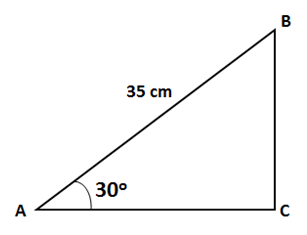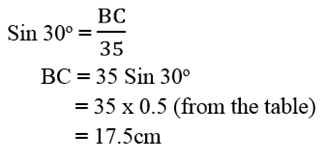# Plane Trigonometry

Trigonometry is the study of relationships between the sides and angles of a triangle. In the following figure, each angle is labelled with a capital letter and the corresponding sides with a lower case letter. As it revolves, it can be imagined that a right angled triangle ABC is formed with the right angle at C. The arm across the right angle is referred as hypotenuse.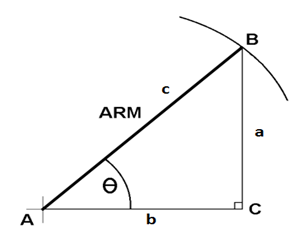The three basic trigonometric functions that involve the angle θ and the triangle sides are sine (sin), cosine (cos) and tangent (tan). The ratios comparing two triangle sides are given below.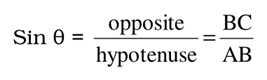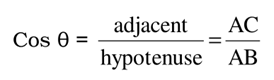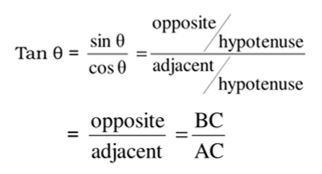Apart from using a calculator, trigonometric table is also used to find out the degree values. A part of the table is shown below.Example

Find BC for the following right angled triangle.